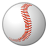# QlikView App Dev

Discussion Board for collaboration related to QlikView App Development.

Announcements
Join us at the Cloud Data and Analytics Tour! REGISTER TODAY
cancel
Showing results for
Search instead for
Did you mean:Contributor

## group by or cluster?

Hello,

I've got a problem with clustering data. I've got dates and orders and I want to know in the first steps, how many orders I've got per day - this works. In the second step, I want to know how many days I've got with 1-5 orders, 6-10 orders and so on.

It shall look like this:I attached the .qvw

Thanks in advance,

Clemens

1 Solution

Accepted SolutionsNot applicable

ok try this:

Load Script:

Orders:

LOAD order,

date,

article

FROM

C:\test.xls

(biff, embedded labels, table is Sheet1\$);

Summary:

Left Join(Orders)

Load date,

if(count(distinct order)<=5,

'days with 1 to 5 orders',

if(count(distinct order)<=10,

'days with 6 to 10 orders',

if(count(distinct order)<=20,

'days with 11 to 20 orders',

'days with >20 orders'))) as  DayType

resident Orders group by date;

Table:

DayType as dimension

count(distinct date) as Expression

11 RepliesContributor
Author

here is the picture:Not applicable

try

if(count(distinct order)<=5,'5',if(count(distinct order)<=10,'10','Other')) as dimensionContributor
Author

thank you, but that is not what I mean. I need the "next step"

15 times <= 5

11 times <=10

...Not applicable

if(count(distinct order)<=5,'5',if(count(distinct order)<=10,'10',if(count(distinct order)<=20,'20','>20')))) as dimensionNot applicable

or:

if(count(distinct order)<=5,'Days with 1 to 5 orders',if(count(distinct order)<=10,'Days with 6 to 10 orders ',if(count(distinct order)<=20,'Days with 11 to 20 orders','days with >20 orders')))) as dimensionContributor
Author

it doesn't work. have you got the .qvw?Not applicable

ok try this:

Load Script:

Orders:

LOAD order,

date,

article

FROM

C:\test.xls

(biff, embedded labels, table is Sheet1\$);

Summary:

Left Join(Orders)

Load date,

if(count(distinct order)<=5,

'days with 1 to 5 orders',

if(count(distinct order)<=10,

'days with 6 to 10 orders',

if(count(distinct order)<=20,

'days with 11 to 20 orders',

'days with >20 orders'))) as  DayType

resident Orders group by date;

Table:

DayType as dimension

count(distinct date) as ExpressionContributor
Author

that`s it! thank you so much!Contributor
Author

Hello,

one more question: is it possible to intricate more than 7 if-formulas? If not, I think I`ll need another solution for my problem...

Thanks,

regards,

cs